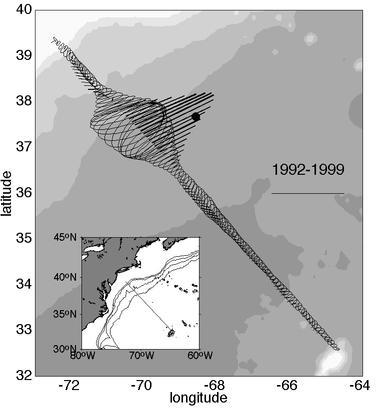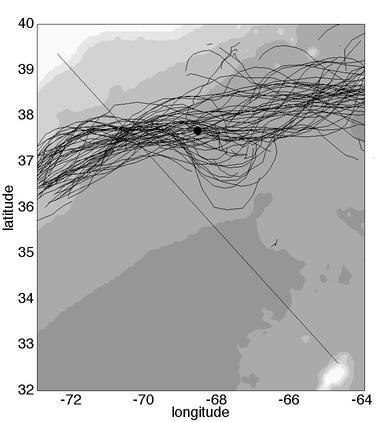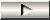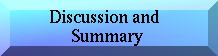Near-surface velocity and PV structure of the Gulf Stream
Abstract >> Introduction >> Data >> Velocity Structure >> Potential Vorticity Structure >>Eddy Exchange Processes >> Discussion and Conclusions

Eddy Exchange Processes

 Here we examine the exchange of momentum and heat between the Gulf Stream and the waters to the north where we have a substantial amount of concurrent XBT data.
• We estimate these covariances in Eulerian coordinates, since so much of the variance results from the meandering of the relatively rigid structure of the current.
• <v'u'> and <v'h'> are estimated with the primes referring to departures from the corresponding local ensemble mean.
• U and V representing down- and cross-stream velocity.
• The x-direction points in the mean downstream direction of 62°T.
• The y-direction points to 332°T.
• The correlation coefficients are about the same, between -0.3 and -0.4 for both momentum and thickness fluxes just north of the Gulf Stream.
• The negative correlations mean that the eddy field is converting eddy momentum and thickness back into the mean flow at this site; the current gains both momentum and thickness from the Slope Sea.
• One way to see this is to consider layer thickness, which decreases monotonically across the Gulf Stream from south to north. A negative correlation <v'h'> north of the stream means that when v'<0,h'>0 and thus the meandering is pushing a thick perturbation south towards where h is thicker. The meandering is 'pumping' thickness fluctuations upgradient.
 Similarly, the orientation of the variance ellipses towards the current from both sides in Figure 1 results from a decreasing meander envelope in the downstream direction forcing the meander-induced eddy field back towards the mean path of the current (Starr, 1968).Figure 1. The Eulerian mean field and variance ellipses at 52 m depth from >300 sections across the NW Atlantic. The maximum velocity vector is 500 km from New Jersey. The direction of mean flow is 65°. The solid dot indicates the location of the H4 mooring in SYNOP (37° 40'N 68° 35'W). The scale to the right indicates 1 m/s and 1 J/kg. Bathymetry is indicated in 1000 m increments.

 A convenient method to get a good measure of the meandering is to examine the trajectories of subsurface floats in the Gulf Stream. Various paths of the Gulf Stream inferred from the trajectories of 68 isopycnal RAFOS floats drifting on/near the 26.8 sigma-t surface (Song et al., 1995). There is a clear convergence of paths as they approach the Oleander line and the pattern of sharp spreading farther east. The ship crosses the current virtually where the path crossings have least spread! This convergence of paths reflects the closing of the meander envelope. We think this is the cause of the apparent conversion of momentum and heat back into the mean flow. Farther to the east, at the black dot, observations show just the opposite, a strong conversion from the mean flow into the eddy field (Johns et al., 1995). Clearly these processes can be quite site-specific.Figure 14. Paths of the Gulf Stream (15°C at 380 m) constructed from 68 isopycnal RAFOS floats on the 26.8 sigma-t-surface. The line indicates the path of the Oleander route. We compute the location where the sloping 15° C (sigma-t = 26.8) surface intersects 380 m (close to the velocity maximum on this surface) using the hyperbolic tangent function: y(Z15) = -40tanh((Z15-380)/300). Given a float's depth, geographical position, direction of motion, we know where it is on this surface and therefore can estimate the normal distance to and hence the position of the intersection of 26.8 sigma-t and 380 m depth.